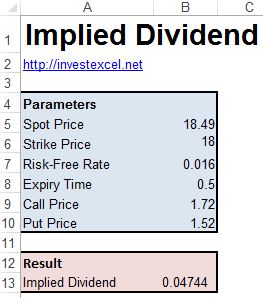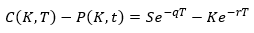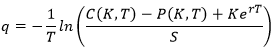# Implied Dividend Calculator

This article teaches you how to calculate the implied dividend of an option via put-call parity, illustrated with an Excel spreadsheet.

Although option holders do not receive dividends, they keenly watch dividend announcements.

Before an announcement, option and stocks are priced to reflect expected dividend payments; however, after dividends are announced, option and stock prices reflect the actual dividend.

The implied dividend is simply the market forecast about the amount of a dividend payment; it essentially captures the opinion of every single trader.You can back out implied dividend through put-call parity. Assuming a European-style option, put-call parity yields the following formula.Rearranging gives this formula.• S is the spot price
• P(K,T) is the price of a put with strike K and maturity T
• C(K,T) is the price of a call with strike K and maturity T
• K is the strike price
• T is the time to maturity
• q is the implied dividend

This Excel spreadsheet implements the above equation.

Download Excel Spreadsheet to Calculate Implied Dividend of an Option### 2 thoughts on “Implied Dividend Calculator”

1. Great website – I’ve been following this website for quite a while. Is q implied dividend a dividend amount (\$) or dividend yield (%)? I can’t seem to tell.